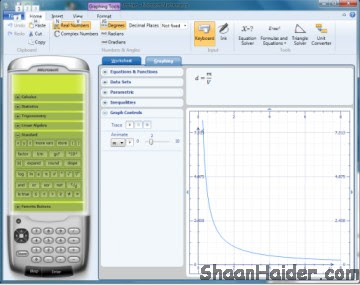# Microsoft Mathematics 4.0 : Free App For Students

If you are one of them who love or hate maths but somehow work much on mathematics then Microsoft is here to help you with a nice free education software Microsoft Mathematics 4.0

Also Check : 101 Facebook Apps For Students

40 Useful Twitter Feeds For College Students

Microsoft Mathematics provides a graphing calculator that plots in 2D and 3D which really helps in understand things easily. step-by-step equation solving, and useful tools to help students with math and science studies.Microsoft Mathematics provides a set of mathematical tools that help students get school work done quickly and easily. With Microsoft Mathematics, students can learn to solve equations step-by-step while gaining a better understanding of fundamental concepts of various branches of mathematics like pre-algebra, algebra, trigonometry, physics, chemistry, and calculus.

Microsoft Mathematics includes a full-featured graphing calculator that’s designed to work just like a handheld calculator. Additional math tools are available in Microsoft Mathematics to help you evaluate triangles, convert from one system of units to another, and solve systems of equations.